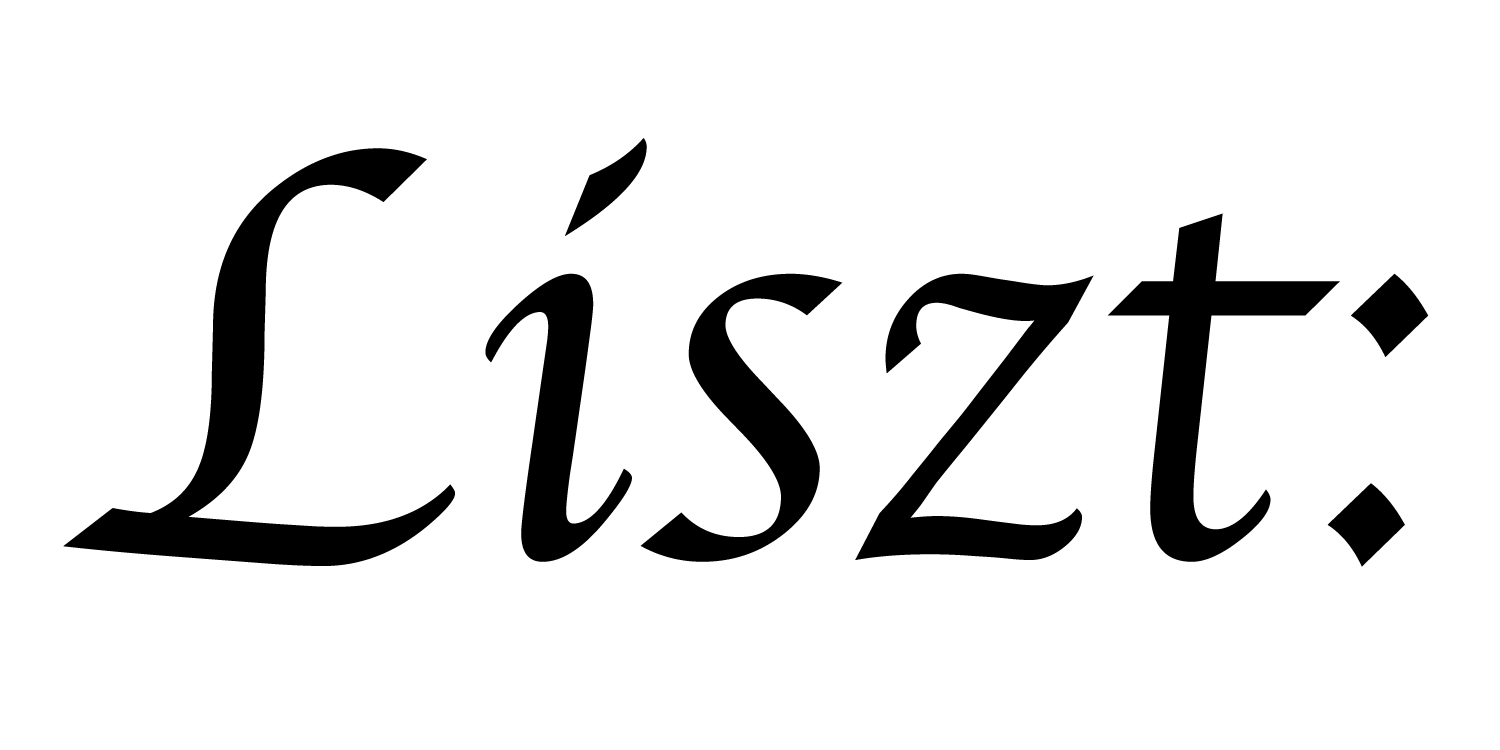# 08: Relations

Grids and Triangle Meshes are both useful geometric domains to be able to compute over, but they’re hardly exhaustive. What if you want to compute over a tetrahedral mesh? A less structured mesh of hexahedral elements? A polygon mesh with polygons of different numbers of sides? A particle system?

The key idea behind Ebb is that a user can implement new geometric domains as re-usable libraries on top of a common relational data model. While most simulation programmers don’t need to understand this relational model, understanding a little bit about relations can help reinforce the ideas from previous tutorials.

In this tutorial, we’ll implement heat diffusion on a torus. But rather than load a triangle-mesh or use the built in grid library, we’ll build our geometric domain from scratch.

``````import 'ebb'
local L = require 'ebblib'

local vdb   = require('ebb.lib.vdb')
``````

We’ll start the program in the usual way

``````local N = 36 -- number of latitudes
local M = 48 -- number of meridians

local vertices  = L.NewRelation {
name = 'vertices',
size = N*M,
}
``````

We can create a new relation with a library call. Relations are like spreadsheet tables, where `size` specifies the number of rows.

All of the “sets of elements” we’ve seen in previous tutorials, including vertices, edges, triangles, and cells are all really just relations. All of our Ebb functions are executed once “for each” row of these relational tables.

``````vertices:NewField('up',     vertices)
vertices:NewField('down',   vertices)
vertices:NewField('left',   vertices)
vertices:NewField('right',  vertices)
``````

In order to connect the vertices to themselves, we’re going to create fields (i.e. columns of the spreadsheet) that hold keys to a relation rather than primitive values like `L.double`. These key-fields are the simplest way to connect relations together. To keep things simple, we don’t separately model the concept of edges here, so we’ve just had these neighbor references direclty refer back to the `vertices` relation.

``````local up_keys     = {}
local down_keys   = {}
local left_keys   = {}
local right_keys  = {}
for i=0,N-1 do
for j=0,M-1 do
up_keys[ i*M + j + 1 ]    = ((i-1)%N) * M + (j)
down_keys[ i*M + j + 1 ]  = ((i+1)%N) * M + (j)
left_keys[ i*M + j + 1 ]  = (i) * M + ((j-1)%M)
right_keys[ i*M + j + 1 ] = (i) * M + ((j+1)%M)
end
end
``````

However, we still need to specify what that connectivity is. Rather than specifying connectivity in the Ebb language, we rely on Lua (or Terra) code to handle data initialization / loading. Here, we first compute and store the indices for each field of keys (0-based) into a corresponding Lua list (1-based indexing).

``````vertices:NewField('pos',L.vec3d)

local vertex_coordinates = {}
for i=0,N-1 do
for j=0,M-1 do
local ang_i = 2 * math.pi * (i/N)
local ang_j = 2 * math.pi * (j/M)
local r, z = math.cos(ang_i) + 1.5, math.sin(ang_i)
local x, y = r*math.cos(ang_j), r*math.sin(ang_j)
vertex_coordinates[ i*M + j + 1 ] = { x, y, z }
end
end

``````

Since we’re thinking of these vertices as living on the surface of a torus, we compute and store the position of each vertex. Just like with the key-fields, this is a Lua computation, so we can’t expect Ebb to accelerate or parallelize it. This is probably ok for some simple examples, but we should remember that this could become a bottleneck as our geometric domain grows.

``````local timestep    = L.Constant(L.double, 0.45)
local conduction  = L.Constant(L.double, 1.0)
local max_diff    = L.Global(L.double, 0.0)

local function init_temperature(idx)
if idx == 0 then return 700 else return 0 end
end

local ebb visualize ( v : vertices )
vdb.color({ 0.5 * v.t + 0.5, 0.5-v.t, 0.5-v.t })
vdb.point(v.pos)
end
``````

Unsurprisingly, much of the setup for a heat diffusion is exactly the same as before.

``````local ebb update_temperature ( v : vertices )
var avg_t   = (v.up.t + v.down.t + v.left.t + v.right.t) / 4.0
var diff_t  = avg_t - v.t
v.new_t     = v.t + timestep * conduction * diff_t
end
``````

We’ll need to modify the update step to use the connectivity relationships we established above.

``````local ebb measure_max_diff( v : vertices )
var avg_t   = (v.up.t + v.down.t + v.left.t + v.right.t) / 4.0
var diff_t  = avg_t - v.t
max_diff max= L.fabs(diff_t)
end
``````

And similarly for measuring our progress so far.

``````for i=1,360 do
vertices:foreach(update_temperature)
vertices:Swap('t', 'new_t')

vdb.vbegin()
vdb.frame()
vertices:foreach(visualize)
vdb.vend()

if i % 10 == 0 then -- measure statistics every 10 steps
max_diff:set(0)
vertices:foreach(measure_max_diff)
print( 'iteration #'..tostring(i), 'max gradient: ', max_diff:get() )
end
end
``````

The simulation loop remains largely the same.

Apart from our use of a visualization library, this heat diffusion program is entirely contained in this one file. So now the domain libraries are a bit more demystified. Most importantly, we know that we can think of all the data in Ebb as being arranged in spreadsheet tables, with a row for each element and a column for each field. This model applies equally well to grid data, although we’ll leave the details of how to build grid-like geometric domains to further optional tutorials. Though even if you’re not interested in learning how to build geometric domain libraries, this basic relational model will help explain Ebb’s more advanced features.

The remaining tutorials are mostly independent of each other. Depending on your expected use cases, you may be able to get started with your own code now and come back to the other tutorials when you get more time.

a part of the Liszt project and PSAAP II center at Stanford University